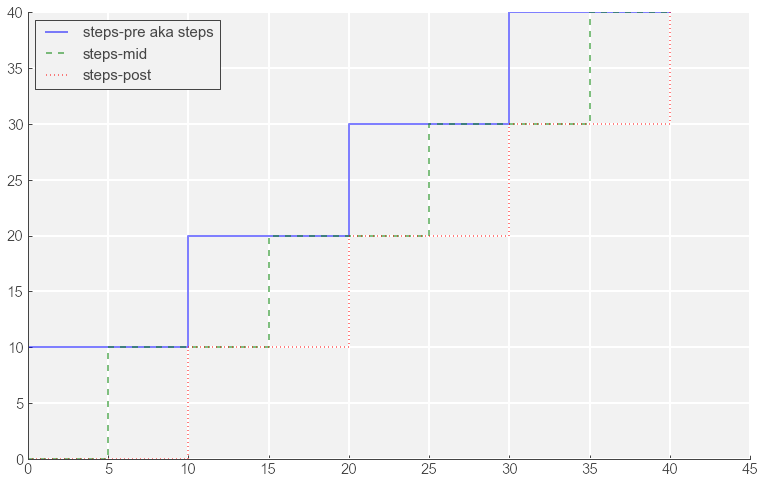# Step Functions in Matplotlib

November 11, 2014      Stardate: 68325.8     Tagged as: Python Matplotlib

This week I had to make a “burn chart”, essentially a chart showing amount of work per week. Since the weeks are discrete time steps I need a step function. I started playing with horizontal and vertical lines but luckily I quickly found that matplotlib has a step function. Unfortunately, it didn’t quite act as I thought.

There are there options for the drawstyle:

1. steps”, which is the same as “steps-pre”
2. steps-mid”
3. steps-post”

Assume the x-vector and y-vector for plotting is

X = [x1, x2, x3, x4]
Y = [y1, y2, y3, y4]

steps

• In this option y2 starts at x1, y3 starts at x2, y4 starts at x3 and ends at x4.

steps-mid

• In this option y1 starts at x1, y2 starts at mid-point between x1 and x2, y3 starts at mid-point between x2 and x3, y4 starts at mid-point between x3 and x4.

steps-post

• In this option y1 starts at x1, y2 starts at x2, y3 starts at x3, y4 starts at x4.

For me, ‘steps-post’ makes the most sense to understand. Below is code to illustrate the options.

In :
%matplotlib inline
from matplotlib import pylab as plt

In :
# make some date
x =  [0, 10, 20, 30, 40]
y =  [0, 10, 20, 30, 40]

# disregard, this is my personal "make pretty" function
fig, ax = myfig()

# plot out the options
plt.plot(x, y, drawstyle='steps', linestyle='-', label='steps-pre aka steps', alpha=0.5,)
plt.plot(x, y, drawstyle='steps-mid', linestyle='--', label='steps-mid', alpha=0.5,)
plt.plot(x, y, drawstyle='steps-post', linestyle=':', label='steps-post', alpha=0.5,)
plt.xlim([0,45])
plt.legend(loc='upper left')
plt.show()In :
%reload_ext version_information
%version_information ipython, pandas, matplotlib

Out:
SoftwareVersion
Python2.7.5+ (default, Feb 27 2014, 19:39:55) [GCC 4.8.1]
IPython2.0.0-dev
OSposix [linux2]
ipython0.13.2
pandas0.13.1
matplotlib1.2.1
Tue Nov 11 22:08:24 2014 PST